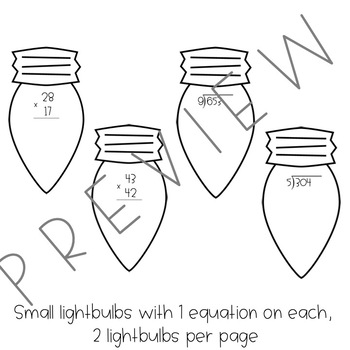# Multiplication & Division [December Bulletin Board]Resource Type
File Type

PDF

(101 MB)
Product Rating
Standards
• Product Description
• StandardsNEW

Tis' the season of lights! Light up your classroom with multiplication & division to celebrate Christmas, Hanukkah and Kwanzaa!

Students will solve various math equations on lightbulbs to be displayed as a string of lights! Print out one light bulb (or many) for each student to complete. When students are finished, hole punch a hole at the top of the light bulb. Use yarn/string to attach the light bulbs. Hang on a bulletin board or in your classroom to light up your room for the holidays!

THIS RESOURCE INCLUDES:

➀ 10 large light bulbs with ➀ 2-digit by 2-digit multiplication equation

➁ 10 large light bulbs with ➁ 2-digit by 2-digit multiplication equations

➂ 10 small light bulbs with ➀ 2-digit by 2-digit multiplication equation on each

➃ 10 large light bulbs with ➀ long division equation

➄ 10 large light bulbs with ➁ long division equations

➅ 10 small light bulbs with ➀ long division equation on each

Happy Christma-hana-kwanzika & Happy Learning ☺️

Find whole-number quotients and remainders with up to four-digit dividends and one-digit divisors, using strategies based on place value, the properties of operations, and/or the relationship between multiplication and division. Illustrate and explain the calculation by using equations, rectangular arrays, and/or area models.
Multiply a whole number of up to four digits by a one-digit whole number, and multiply two two-digit numbers, using strategies based on place value and the properties of operations. Illustrate and explain the calculation by using equations, rectangular arrays, and/or area models.
Total Pages
N/A
N/A
Teaching Duration
N/A
Report this Resource to TpT
Reported resources will be reviewed by our team. Report this resource to let us know if this resource violates TpT’s content guidelines.